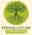Language

# How Much Potting Mix Does a Garden Pot Hold?2 months ago 127
FAIRE UN DON URGENT QuebecNouvelles.com & ParoleJuste.comPlastic garden pots come in a range of standard sizes. When filling a large pot, or a number of smaller pots, gardeners often have to estimate how much potting mix they will require.

Bags of potting mix specify how many litres they hold, usually 25-30L for a standard bag, and 40L in the extra-large ones. But what about pots, where do we find their volume?

When referring to pot sizes, pots are measured by their width across the top. So, a pot measuring 15cm (6”) across the top is referred to as a 15cm or 6” pot. This measurement, and sometimes figure for the volume, may be printed on the bottom of plastic pots.The width measurement doesn’t account for the height, and a taller pot of the same width will obviously hold much more. The way the horticulture industry gets around this is to use standardised sizes of pots, so a standard pot of a certain width will be a particular height. Lower height pots are referred to as squat pots, to distinguish them for the regular standard pots.

Using the table below, it’s easy to figure out how much potting mix is needed to fill a standard pot.

Pot width     Pot Volume

10cm (4“)  –  0.5 L (0.13 gal)

15cm (6“)  –  1.9 L (0.50 gal)

20cm (8“)  –  4.5 L (1.19 gal)

25cm (10“) – 8L (2.11 gal)

30cm (12“) – 13.5L (3.57 gal)

33cm (13“) – 18L (4.75 gal)

42cm (16“) – 28L (7.40 gal)

50cm (20“) – 52L (13.75 gal)

If we have a 25L bag of potting mix, and are filling 15cm (6”) pots which hold 1.9L of potting mix, we can fill 25/1.9 = 13.16 or 13 pots.

## What is the Correct Level to Fill Garden Pots?When filling pots with potting mix, they should never filled to the top. Smaller pots are usually filled at least 1cm (3/8”) below the top. With larger pots, leave a space of 2cm (3/4″) or more.

This empty space makes watering easier, and prevents the potting mix and any fertiliser applied to the pot washing out.

When watering, fill the empty space at the top of the pot with water, all the way to the brim. That’s what it’s for! Let the water drain down into the potting mix. Repeat if necessary, until the water just begins to trickle out from the drainage holes at the bottom, to ensure the potting mix is watered evenly, all the way through.

## How to Calculate the Volume of a Garden Pot

What can we do if we have a non-standard sized pot that we need to know the volume of? The volume of any traditionally shaped pot can be calculated mathematically. To do this, all we need to do is measure the width at the top and bottom of the pot, and its height.

A regular shaped pot has a round top, with a smaller round base, and sloped sides. This shape is a an inverted cone with the pointed end removed. The correct name for this shape is a truncated cone, also known as a frustum of a cone or conical frustum, a cone which is sliced somewhere along its length parallel to the base.

The diagram below shows the truncated cone shape of a typical garden pot.The formula to calculate the volume of truncated cone:

Volume (V) = 1/3 * π * h(r^2 + R^2 + r*R)

where

• r = radius of smaller circle (half the width of the base)
• R = radius of bigger circle (half the width of the top)
• h = height of the truncated cone
• π = pi = 22/7 = 3.14286

For those not so mathematically inclined, we can express the formula in a simpler way:

Volume (V) = 1/3 x 22/7 x h x (rxr + RxR + rxR)

If we’re putting the formula into a spreadsheet, we can enter it as =1/3*22/7*C2*(B2^2+A2^2+B2*A2)

where

• The value of R is in cell A2
• The value or r is in cell B2
• The value of h is in cell C2Since our values are in cm, the answer is in cubic centimetres, which is the same as mL (millilitres), in this case 639ml. If our values are in inches, then the final volume calculated will be in cubic inches.

Note: Remember that we shouldn’t fill pots all the way to the top, so when calculating the volume, reduce the height value h by the amount of centimetres or inches of space that we wish to allow at the top of the pot.

It’s much easier to purchase the right amount of potting mix when we know how much our pots can hold. Using the table or the formula above, we can calculate the volume of any traditionally shaped pot.